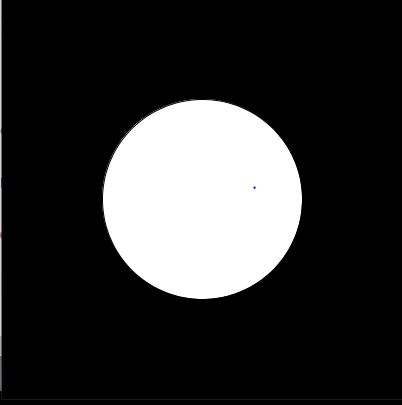Open in App
Not now

# Introduction to Processing | Java

• Difficulty Level : Medium
• Last Updated : 07 May, 2020

Processing is an open-source low level animation and GUI library built on Java with additional simplifications like additional classes, aliased mathematical functions and operations. It also provides a GUI for simple compilation of the programs written in processing.

Features of Processing: The following are the features of processing:

• It includes a sketchbook which is a minimalistic alternative to an IDE. This sketchbook can be used as a normal IDE to organize projects.
• Every sketch drawn in processing is a subclass of the Java class(PApplet). This class implements almost all the features of processing.
• Since processing inherits the properties of the class, all the additional classes defined in the sketch will be treated as an inner class when the code is being converted into a pure java code before compiling. Therefore, the use of static variables and methods is strictly prohibited in processing.
• The processing language also gives the users an option to create own classes in the PApplet sketch. Therefore, this gives the users a chance to use a more complex data structures apart from the basic data types in java.

Installing Processing: In order to code in the processing language, the users can either download processing sketchbook from the official website. Apart from that, the users can also download the code jar file and set it up in any of the IDE to use processing.

## Recommended: Please try your approach on {IDE} first, before moving on to the solution.

Example: The following is an example to get an understanding of how to code in processing. Let’s see how to draw a circle in processing. In order to do this, we need to learn about the main function that processing invokes from its library. That means, we only have to define this function but not invoke it.

Below is a sample processing code which draws a circle:

 `// This function is called whenever we``// start the app.``void` `setup()``{``    ``// This function is a built in function``    ``// in processing which takes two``    ``// arguments: width and height``    ``// 400, 400 means a window of``    ``// length 400 pixels and width``    ``// 400 pixels``    ``size(``400``, ``400``);``}`` ` `// This function is called once per``// frame. That is, if the frame rate``// is 60, then this will be called``// 60 times in one second``void` `draw()``{`` ` `    ``// This is also an inbuilt function``    ``// which can take 4, 3 or 1 argument``    ``// where each argument represents the``    ``// intensity of each colour like:``    ``// 4 = (red, green, blue alpha)``    ``// 3 = (red, green, blue)``    ``// 1 = (gray_scale_value)``    ``background(``0``);`` ` `    ``// This command draws the circle``    ``// on our canvas at x=width/2,``    ``// y=height/2, diameter=200``    ``circle(width / ``2``, height / ``2``, ``200``);``}`

Output: The output for the above program is:My Personal Notes arrow_drop_up